## 安装依赖

`pip install pytrends`

`pip install requests[socks]`

## 编写代码

`from pytrends.request import TrendReq`

``````pytrends = TrendReq(hl='en-US', tz=360)
pytrends.build_payload(['Coronavirus'], cat=0, timeframe='2020-02-01 2020-03-10',  gprop='', geo='US-NY')``````

``````df = pytrends.interest_over_time()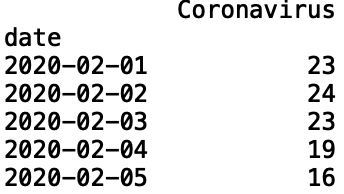``````import matplotlib.pyplot as plt
import seaborn as sns
import pandas as pdsns.set()
df['timestamp'] = pd.to_datetime(df.index)
sns.lineplot(df['timestamp'], df['Coronavirus'])plt.title("Normalized Searches for Coronavirus in NY")
plt.ylabel("Number of Searches")
plt.xlabel("Date")``````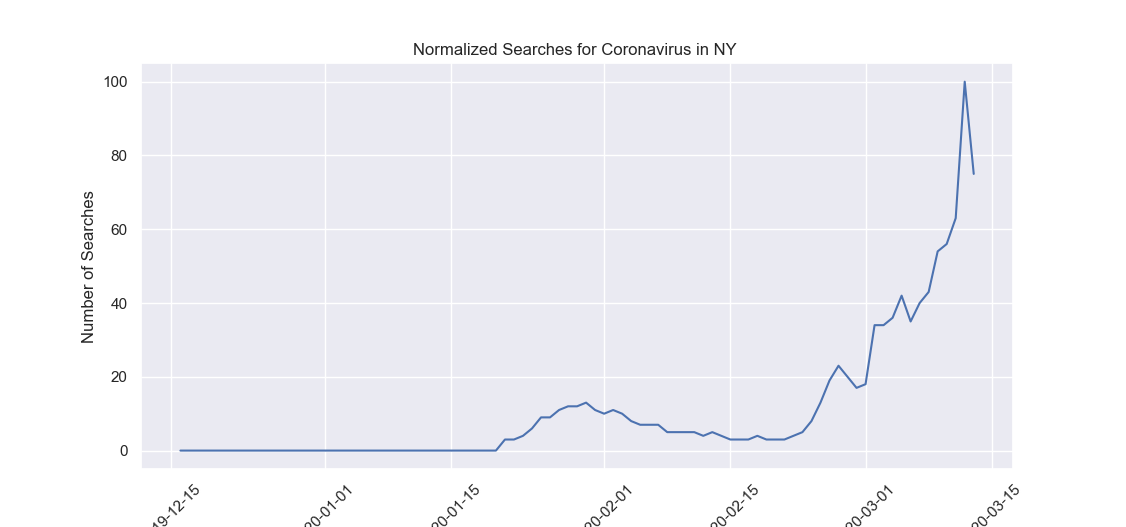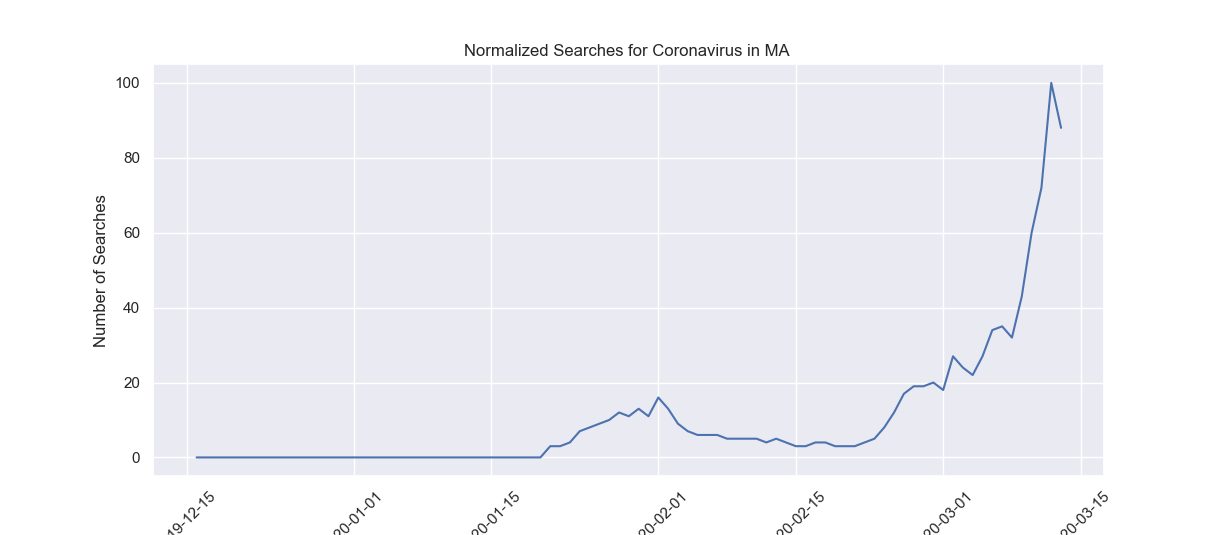## 封装成函数

``````def get_searches(key_word, state):
pytrends = TrendReq(hl='en-US', tz=360)
df = pytrends.interest_over_time()

sns.set()
df['timestamp'] = pd.to_datetime(df.index)
sns.lineplot(df['timestamp'], df[key_word])

plt.title("Normalized Searches for {} in {}".format(key_word, state))
plt.ylabel("Number of Searches")
plt.xlabel("Date")``````

``````get_searches('Coronavirus', 'NY')
get_searches('Coronavirus', 'MA')
get_searches('Coronavirus', 'CA')``````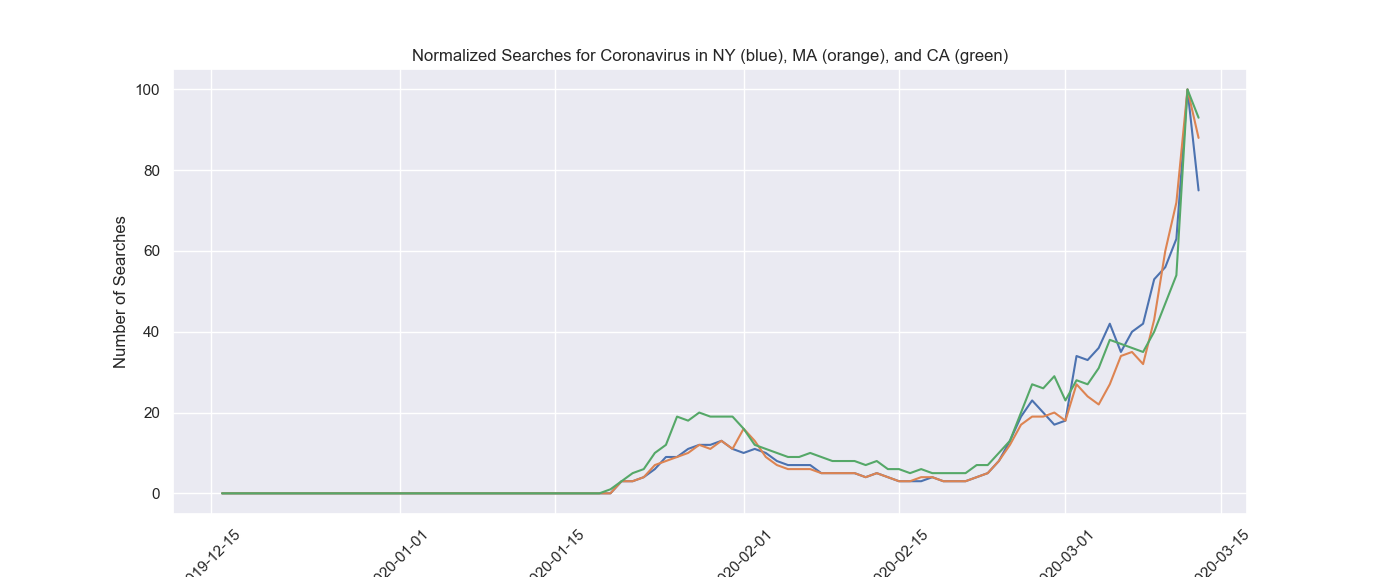## 这里是送的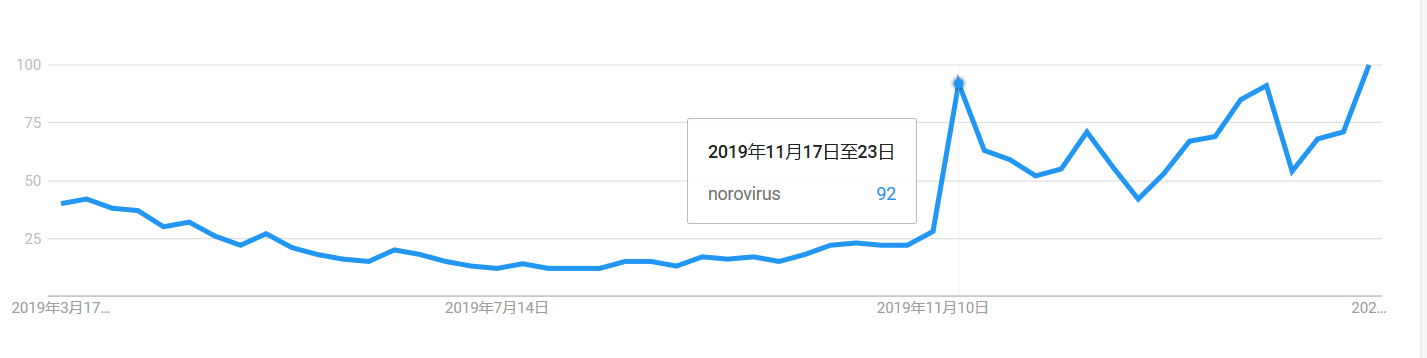###### 评论